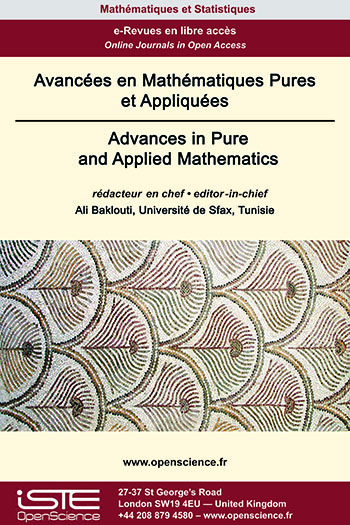# Vol 13 - Issue 3 (June 2022)

## List of Articles

Limit sets and global dynamic for 2-D divergence-free vector fields
Habib Marzougui

The global structure of divergence-free vector fields on closed surfaces is investigated. We prove that if M is a closed surface and ${V}$ is a divergence-free $C^{1}$-vector field with finitely many singularities on M then every orbit L of $\mathcal{V}$ is one of the following types: (i) a singular point, (ii) a periodic orbit, (iii) a closed (non periodic) orbit in M* = M - Sing($\mathcal{V}$), (iv) a locally dense orbit, where Sing($\mathcal{V}$) denotes the set of singular points of $\mathcal{V}$. On the other hand, we show that the complementary in M of periodic components and minimal components is a compact invariant subset consisting of singularities and closed (non compact) orbits in M*. These results extend those of T. Ma and S. Wang in [Discrete Contin. Dynam. Systems, 7 (2001), 431-445] established when the divergence-free vector field $\mathcal{V}$ is regular that is all its singular points are non-degenerate.

Weighted estimates for operators associated to the Bergman-Besov kernels
David Békollè, Adriel R. Keumo, Edgar L. Tchoundja, Brett D. Wick

We characterize the weights for which we have the boundedness of standard weighted integral operators induced by the Bergman-Besov kernels acting between two weighted Lebesgue classes on the unit ball of ℂN in terms of Békollè - Bonami type condition on the weights. To accomplish this we employ the proof strategy originated by Békollè.

A result on Bruck Conjecture related to Shift Polynomials
B. Narasimha Rao, Shilpa N.

This paper mainly concerns about establishing the Bruck conjecture for differential-difference polynomial generated by an entire function. The polynomial considered is of finite order and involves the entire function $f(z)$ and its shift $f(z + c)$ where $c \in ℂ$. Suitable examples are given to prove the sharpness of sharing exceptional values of Borel and Nevanlinna.

Existence Results for Singular p(x)-Laplacian Equation
R. Alsaedi, K. Ben Ali, A. Ghanmi

This paper is concerned with the existence of solutions for the following class of singular fourth order elliptic equations
$\left\{ \begin{array}{ll} \Delta\Big(｜x｜^{p(x)}｜\Delta u｜^{p(x)-2}\Delta u\Big)=a(x)u^{-\gamma (x)}+\lambda f(x,u),\quad \mbox{in }\Omega, \\ u=\Delta u=0, \quad \mbox{on }\partial\Omega. \end{array} \right.$
where $\Omega$ is a smooth bounded domain in $\mathbb{R}^N, \gamma :\overline{\Omega}\rightarrow (0,1)$ be a continuous function, $f\in C^{1}( \overline{\Omega}\times \mathbb{R}), p:\; \overline{\Omega}\longrightarrow \;(1,\infty)$ and $a$ is a function that is almost everywhere positive in $\Omega$. Using variational techniques combined with the theory of the generalized Lebesgue-Sobolev spaces, we prove the existence at least one nontrivial weak solution.### Other issues :

2023

Volume 23- 14

Issue 1 (January 2023)
Issue 2 (Special CSMT 2022)
Issue 3 (June 2023)
Issue 4 (September 2023)

2022

Volume 22- 13

Issue 1 (January 2022)
Issue 2 (March 2022)
Issue 3 (June 2022)
Issue 4 (September 2022)

2021

Volume 21- 12

Issue 1 (January 2021)
Issue 2 (May 2021)
Issue 3 (Special AUS-ICMS 2020)
Issue 4 (September 2021)

2020

Volume 20- 11

Issue 1 (May 2020)
Issue 2 (September 2020)